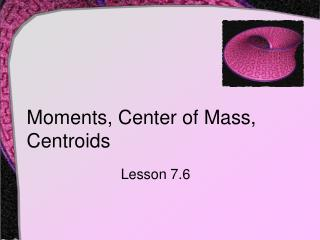DownloadDownload PresentationMoments, Center of Mass, Centroids

# Moments, Center of Mass, Centroids

Download Presentation## Moments, Center of Mass, Centroids

- - - - - - - - - - - - - - - - - - - - - - - - - - - E N D - - - - - - - - - - - - - - - - - - - - - - - - - - -
##### Presentation Transcript

1. Moments, Center of Mass, Centroids Lesson 7.6

2. Mass • Definition: mass is a measure of a body's resistance to changes in motion • It is independent of a particular gravitational system • However, mass is sometimes equated with weight (which is not technically correct) • Weight is a type of force … dependent on gravity

3. Mass • The relationship is • Contrast of measures of mass and force

4. Centroid • Center of mass for a system • The point where all the mass seems to be concentrated • If the mass is of constant density this point is called the centroid 4kg 10kg 6kg •

5. Centroid • Each mass in the system has a "moment" • The product of the mass and the distance from the origin • "First moment" is the sum of all the moments • The centroid is 4kg 10kg 6kg

6. Also notated Mx,moment about x-axis First moment of the system Also notated My, moment about y-axis Also notated m, the total mass Total mass of the system Centroid • Centroid for multiple points • Centroid about x-axis

7. Centroid • The location of the centroid is the ordered pair • Consider a system with 10g at (2,-1), 7g at (4, 3), and 12g at (-5,2) • What is the center of mass?

8. Centroid • Given 10g at (2,-1), 7g at (4, 3), and 12g at (-5,2) 7g 12g 10g

9. a b Centroid • Consider a region under a curve of a material of uniform density • We divide the region into rectangles • Mass of each considered to be centered at geometric center • Mass of each is the product of the density, ρand the area • We sum the products of distance and mass •

10. Centroid of Area Under a Curve • First moment with respectto the y-axis • First moment with respectto the x-axis • Mass of the region

11. Centroid of Region Between Curves f(x) • Moments • Mass g(x) Centroid

12. Try It Out! • Find the centroid of the plane region bounded by y = x2 + 16 and the x-axis over the interval 0 < x < 4 • Mx = ? • My = ? • m = ?

13. Theorem of Pappus • Given a region, R, in the plane and L a line in the same plane and not intersecting R. • Let c be the centroid and r be the distance from L to the centroid R L r c

14. Theorem of Pappus • Now revolve the region about the line L • Theorem states that the volume of the solid of revolution iswhere A is the area of R R L r c

15. Assignment • Lesson 7.6 • Page 504 • Exercises 1 – 41 EOO also 49